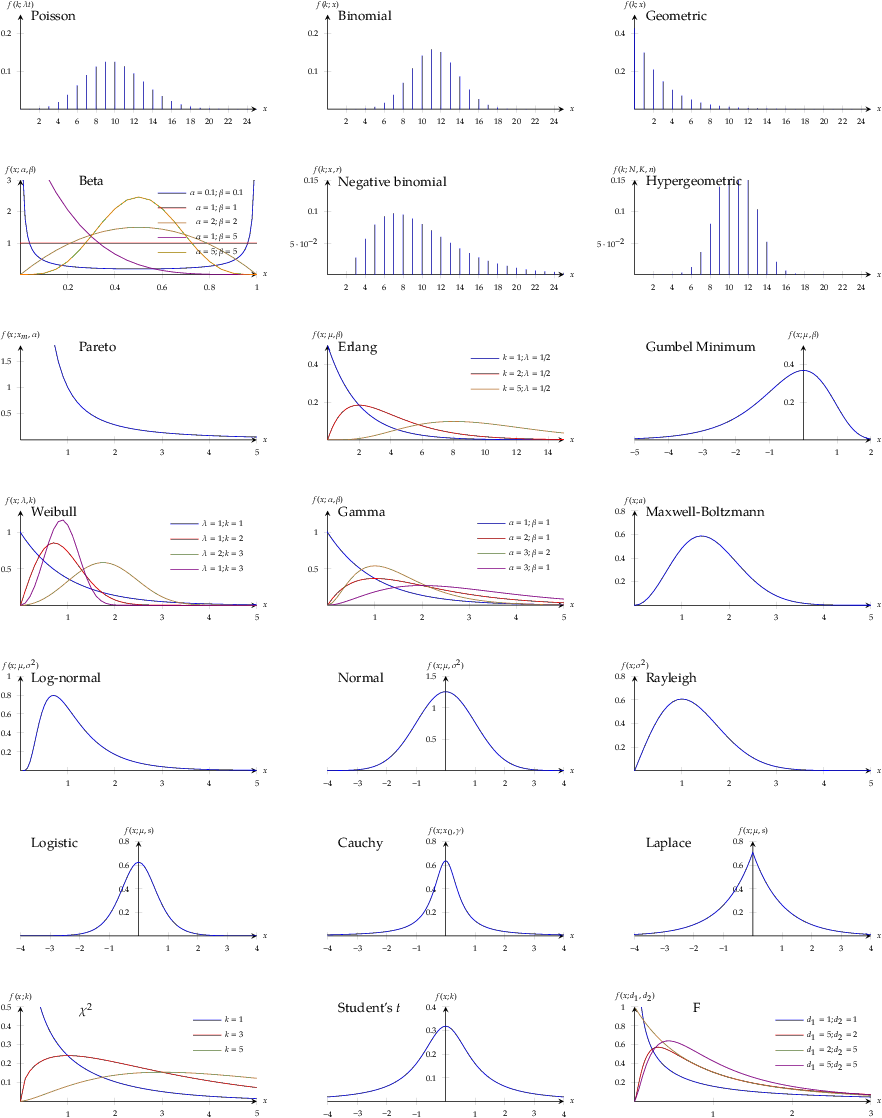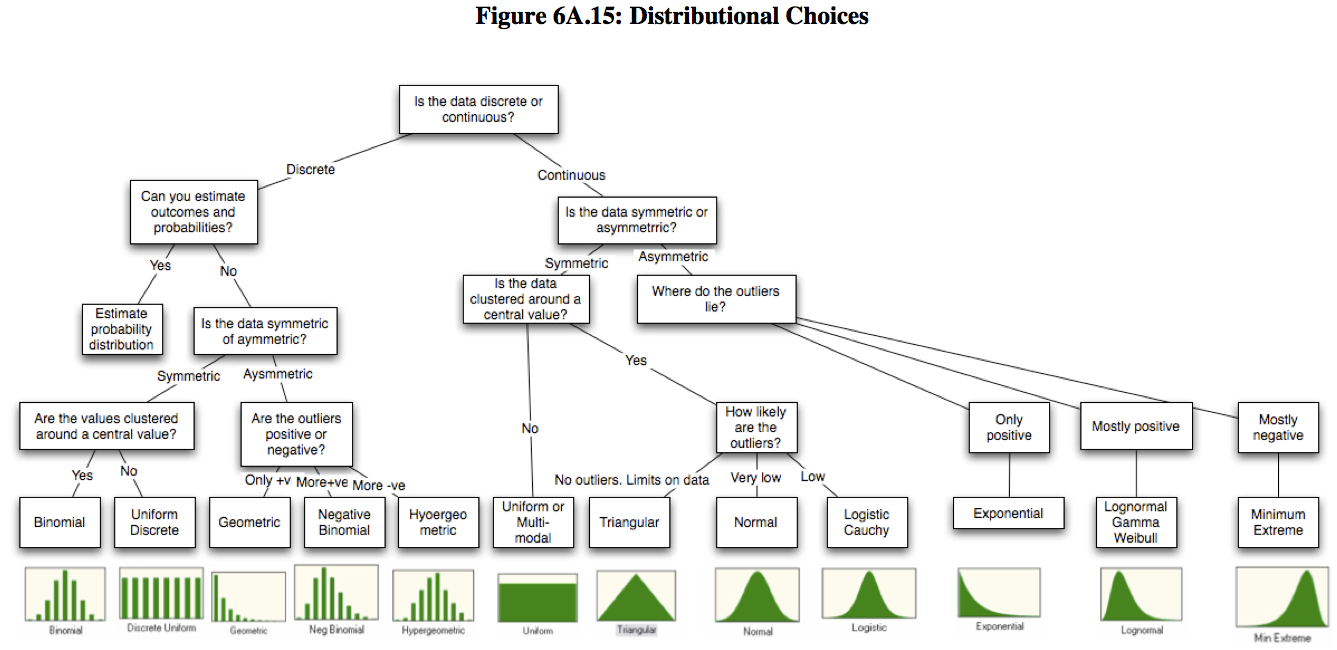This is a summary of common probability distributions in engineering and statistics. This chart has the plots of the pdf or pmf (LaTeX source):# discrete distributions

binomial distribution

• A big urn with balls in either white or black color. Drawing a white ball from urn has probability $$x$$ (i.e., black ball has probability $$1-x$$). If we draw $$n$$ balls from urn with replacement, the probability of getting $$k$$ white balls:
$f(k; n, x) = \binom{n}{k} x^k (1-x)^{n-k}$

Poisson distribution

• Balls are added to the urn at rate of $$\lambda$$ per unit time, under exponential distribution. The probability of having $$k$$ balls added to the urn within time $$t$$:
$f(k; \lambda t) = \frac{(\lambda t)^k e^{-\lambda t}}{k!}$

geometric distribution

• The probability of have to draw $$k$$ balls to see the first white ball being drawn:
$f(k; x) = (1-x)^{k-1} x$

negative binomial distribution

• same as the distribution of the sum of $$r$$ iid geometric random variable
• negative binomial approximates Poisson with $$\lambda = r(1-x)$$ with large $$r$$ and $$x\approx 1$$
• Drawing balls from the urn. If we have to draw $$k$$ balls to see the $$r$$-th white ball (we have drawn $$r$$ white balls and $$k-r$$ black balls). The probability of $$k$$:
$f(k; r, x) = \binom{k-1}{k-r} x^r (1-x)^{k-r}$

hypergeometric distribution

• A urn with $$N$$ balls (finite) and $$K$$ balls amongst are white. Draw, without replacement, $$n$$ balls from the urn to get $$k$$ white balls:
$f(k; N, K, n) = \frac{\binom{K}{k}\binom{N-K}{n-k}}{\binom{N}{n}}$

# continuous distributions

uniform distribution

• extreme of flattened distribution
• with upper and lower bounds

triangular distribution

• with upper and lower bounds

normal distribution

• strong tendency for data at central value; symmetric, equally likely for positive and negative deviations from its central value
• frequency of deviations falls off rapidly as we move further away from central value
$f(x; \mu, \sigma^2) = \frac{1}{\sqrt{2\pi\sigma^2}}\exp(-\frac{(x-\mu)^2}{2\sigma^2})$
• $X_1 \sim N(\mu_1, \sigma^2_1); X_2 \sim N(\mu_2, \sigma^2_2) \to X_1+X_2 \sim N(\mu_1+\mu_2, \sigma_1^2+\sigma_2^2)$
• approximation to Poisson distribution: if $$\lambda$$ is large, Poisson distribution approximates normal with $$\mu=\sigma^2=\lambda$$
• approximation to binomial distribution: if $$n$$ is large and $$x\approx \frac{1}{2}$$, binomial distribution approximates normal with $$\mu=nx$$ and $$\sigma^2=nx(1-x)$$
• approximation to beta distribution: if $$\alpha$$ and $$\beta$$ are large, beta distribution approximates normal with $$\mu=\frac{\alpha}{\alpha+\beta}$$ and $$\sigma^2=\frac{\alpha\beta}{(\alpha+\beta)^2(\alpha+\beta+1)}$$

Laplace distribution

• absolute difference from mean compared to squared difference in normal distribution
• longer (fatter) tails, higher kurtosis (flattened peak)
• pdf:
$f(x; \mu, s) = \frac{1}{2s}\exp(-\frac{|x-\mu|}{s})$

logistic distribution

• symmetric, with longer tails and higher kurtosis than normal distribution
• logistic distribution has finite mean $$\mu$$ and variance defined
• $X\sim U(0,1) \to \mu+s[\log(X)-\log(1-X)] \sim \textrm{Logistic}(\mu,s)$
• $X\sim \textrm{Exp}(1) \to \mu+s\log(e^X-1) \sim \textrm{Logistic}(\mu,s)$
• logistic pdf:
$f(x; \mu, s) = \frac{e^{-(x-\mu)/s}}{s\left(1+e^{-(x-\mu)/s}\right)^2}$

Cauchy distribution

• symmetric, with longer tails and higher kurtosis than normal distribution
• Cauchy distribution has mean and variance undefined, but mean & mode at $$\mu$$
• $X,Y\sim N(\mu,\sigma^2) \to X/Y \sim \textrm{Cauchy}(\mu,\sigma^2)$
• Cauchy pdf:
$f(x; x_0, \gamma) = \frac{1}{\pi\gamma\left[1+\left(\frac{x-x_0}{\gamma}\right)^2\right]}$

lognormal distribution

• $$\log(X)\sim N(\mu,\sigma^2)$$, positively skewed
• parameterised by shape ($$\sigma$$), scale ($$\mu$$, or median), shift ($$\theta$$)
• $$\mu=0, \theta=1$$ is standard lognormal distribution
• as $$\sigma$$ rises, the peak shifts to left and skewness increases
$f(x; \mu, \sigma^2) = \frac{1}{\sqrt{2\pi x^2\sigma^2}}\exp(-\frac{(\log x-\mu)^2}{2\sigma^2})$
• sum of two lognormal random variable is a lognormal random variable with $$\mu=\mu_1+\mu_2$$ and $$\sigma^2=\sigma_1^2+\sigma_2^2$$

Pareto distribution

• power law probability distribution
• continuous counterpart of Zipf’s law
• positively skewed, no negative tail, peak at $$x=0$$
$f(x; x_m, \alpha) = \frac{\alpha x_m^{\alpha}}{x^{\alpha+1}}$

gamma distribution

• support for $$x\in(0,\infty)$$, positive skewness (lean left)
• decreasing $$\alpha$$ will push distribution towards the left; at low $$\alpha$$, left tail will disappear and distribution will resemble exponential
• models the time to the $$\alpha$$-th Poisson arrival with arrival rate $$\beta$$
• gamma pdf ($$\alpha=1$$ becomes exponential pdf with rate $$\beta$$):
$f(x; \alpha, \beta) = \frac{\beta^{\alpha}}{\Gamma(\alpha)} x^{\alpha-1}e^{-\beta x}$

Weibull distribution

• support for $$x\in(0,\infty)$$, positive skewness (lean left)
• decreasing $$k$$ will push distribution towards the left; at low $$k$$, left tail will disappear and distribution will resemble exponential
• If $$W\sim\textrm{Weibull}(k,\lambda)$$, then $$X=W^k \sim \textrm{Exp}(1/\lambda^k)$$
• Weibull pdf ($$k=1$$ becomes exponential pdf with rate $$1/\lambda$$):
$f(x; k, \lambda) = \frac{k}{\lambda}\left(\frac{x}{\lambda}\right)^{k-1}e^{-(x/\lambda)^k}$

Erlang distribution

• $X_i\sim\textrm{Exp}(\lambda) \to \sum_{i=1}^k X_i \sim \textrm{Erlang}(k, \lambda)$
• arise from teletraffic engineering: time to $$k$$-th call
$f(x; k,\lambda) = \frac{\lambda^k x^{k-1} e^{-\lambda x}}{(k-1)!}$

beta distribution

• support for $$x\in(0,1)$$
• allows negative skewness
• two shape parameters $$p$$ and $$q$$, and lower- and upper-bounds on data ($$a$$ and $$b$$)
$f(x; \alpha, \beta) = \left(\frac{\Gamma(\alpha)\Gamma(\beta)}{\Gamma(\alpha+\beta)}\right)^{-1} x^{\alpha-1}(1-x)^{\beta-1}$

extreme value distribution (i.e. Gumbel minimum distribution)

• negatively skewed
• Gumbel maximum distribution, $$f(-x;-\mu,\beta)$$, is positively skewed
• Limiting distribution of the max/min value of $$n\to\infty$$ iid samples from $$\textrm{Exp}(\lambda)$$ with $$\lambda = 1/\beta$$
• standard cdf: $$F(x)=1-\exp(-e^x)$$
$f(x; \mu, \beta) = \frac{1}{\beta}e^{(\mu-x)/\beta}e^{-e^{(\mu-x)/\beta}}$

Rayleigh distribution

• positively skewed
• modelling the $$L^2$$-norm of two iid normal distribution with zero mean (e.g., orthogonal components of a 2D vector)
$f(x; \sigma) = \frac{x}{\sigma^2} \exp(-\frac{x^2}{2\sigma^2})$

Maxwell-Boltzmann distribution

• positively skewed
• 3D counterpart of Rayleigh distribution
• arise from thermodynamic: probability of a particle in speed $$v$$ if temperature is $$T$$
\begin{align} f(v; \sqrt{kT/m}) &= \left(\frac{m}{2\pi kT} \right)^{3/2} 4\pi v^2 \exp(-\frac{mv^2}{2kT}) \\ f(x; a) &= \sqrt{\frac{2}{\pi}}\frac{x^2 e^{-x^2/(2a^2)}}{a^3} \end{align}

Chi-squared distribution

• distribution of the sum of the square of $$k\ge 1$$ i.i.d. standard normal random variables
• mean $$k$$, variance $$2k$$
• PDF with $$k$$ degrees of freedom:
$f(x; k) = \frac{x^{k/2-1} e^{-x/2}}{2^{k/2}\Gamma(k/2)}$

F-distribution

• Distribution of a random variable defined as the ratio of two independent $$\chi^2$$-distributed random variables, with degrees of freedom $$d_1$$ and $$d_2$$ respectively
• Commonly used in ANOVA
• PDF, with degrees of freedom $$d_1$$ and $$d_2$$, involves beta function $$B(\alpha,\beta)$$:
\begin{align} f(x; d_1, d_2) &= \left[x B(\frac{d_1}{2},\frac{d_2}{2})\right]^{-1} \sqrt{\frac{(d_1 x)^{d_1} d_2^{d_2}}{(d_1 x+d_2)^{d_1+d_2}}} \\ \textrm{and if}\qquad z_1 &\sim \chi^2(d_1) \\ z_2 &\sim \chi^2(d_2) \\ \textrm{then}\qquad x = \frac{z_1/d_1}{z_2/d_2} &\sim f(x, d_1, d_2) \end{align}

Student’s t distribution

• Distribution of normalized sample mean of $$n=k+1$$ observations from a normal distribution, $$\frac{\bar{X}-\mu}{S/\sqrt{n}}$$
• Equivalently, this is the distribution of $$\frac{x}{\sqrt{y/r}}$$ for $$x$$ is standard normal and $$y$$ is chi-square with $$r$$ degrees of freedom
• t distribution with $$n=1$$ is Cauchy distribution
• PDF with degree of freedom $$k$$:
$f(x, k) = \frac{\Gamma(\frac{k+1}{2})}{\sqrt{k\pi}\Gamma(k/2)} \left(1+\frac{x^2}{k}\right)^{-(k+1)/2}$

# test of fit for distributions

Kolmogorov-Smirnov test (K-S test, on cumulative distribution function $$F(x)$$)

$D_n = \sup_x | F_n(x) - F(x) |$
• if sample comes from distribution, $$D_n$$ converges to 0 a.s. as number of samples $$n$$ goes to infinity

Shapiro-Wilk test

$W = \frac{\sum_{i=1}^n a_i x_i}{\sum_{i=1}^n (x_i - \bar{x})^2}$
• test of normality in frequentist statistics (i.e. for $$x_i$$ in normal distribution)
• $$\bar{x} = \frac{1}{n}(x_1 + \cdots + x_n)$$ is the sample mean
• $$(a_1,\cdots,a_n) = m^T V^{-1} (m^T V^{-1}V^{-1} m)^{-1/2}$$ where $$m$$ is vector of expected values of the order statistics from normal distribution and $$V$$ the covariance matrix of those order statistics

Anderson-Darling test

$A^2 = n \int_{-\infty}^{\infty} \frac{(F_n(x)-F(x))^2}{F(x)(1-F(x))} dF(x)$
• test whether a sample comes from a specified distribution
• $$A^2$$ is weighted distance between $$F_n(x)$$ and $$F(x)$$, with more weight on tails of the distribution

Pearson’s $$\chi^2$$ test

$\chi^2 = \sum_{i=1}^n \frac{(O_i - E_i)^2}{E_i}$
• test for categories fit a distribution: checking observed frequency $$O_i$$ against expected frequency $$E_i$$ according to distribution for each of $$n$$ categories
• degree of freedom: $$n$$ minus number of parameters of the fitted distribution

# Reference

Lawrence M. Leemis and Jacquelyn T. McQuestion. Univariate Distribution Relationships, Am Stat, 62(1) pp.45–53, 2008, DOI: 10.1198/000313008X270448

Aswath Damodaran. Probabilistic approaches: Scenario analysis, decision trees and simulations (PDF, the appendix is also available separately) and includes the following chart for choosing a distribution: